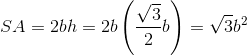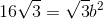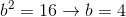# GMAT Math : Calculating the length of an edge of a tetrahedron

## Example Questions

### Example Question #1 : Tetrahedrons

Suppose the volume of the tetrahedron is.  What is the edge of the tetrahedron?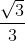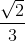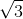Explanation:

Write the formula to solve for the edge of a tetrahedron.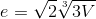Substitute the volume and solve.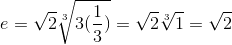### Example Question #2 : Tetrahedrons

The height of one of the equilateral triangle faces on a tetrahedron is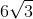.  What is the side length of the tetrahedron?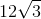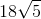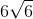Explanation:

Because a tetrahedron has four congruent equilateral triangles as its faces, we know the three equal angles of each face, as with all equilateral triangles, are each.  We are given the height of a face, which is the length of a line that bisects one of theangles and forms a right triangle with the side length as its hypotenuse. This means the angle between the height and the side length is,  whose cosine is equal to the adjacent side, the height, over the hypotenuse, the side length. This gives us: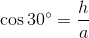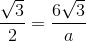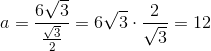### Example Question #3 : Tetrahedrons

The volume of a tetrahedron is. What is the edge of the tetrahedron?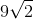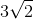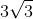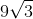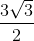Explanation:

Write the formula to solve for the edge of a tetrahedron.Substitute the given volume to the equation and solve.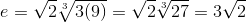### Example Question #4 : Tetrahedrons

Find the exact edge length of a tetrahedron if the volume is.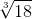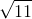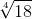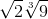Explanation:

Write the tetrahedron formula to solve for the edge.Substitute the volume and simplify.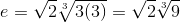### Example Question #5 : Tetrahedrons

A certain tetrahedron has a surface area of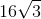.  What is the length of an edge of the tetrahedron?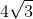Explanation:

A tetrahedron has four congruent equilateral triangle faces, so its surface area is four times the area of one of these equilateral triangles. This gives us: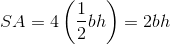Whereis the base of the triangle, or the edge length of the tetrahedron, andis the height of each triangular face. We want to solve for,  but we are only given the surface area and not the height, so we need to express this value in terms of the base. One way of doing this is by recognizing that the height of each equilateral triangle face bisects an angle and forms two congruent right triangles for which it is the base and the edge length, or,  is the hypotenuse. Each angle in an equilateral triangle is,  so if one is bisected by the height then the angle between it and the hypotenuse is.  The cosine of this angle is equal to the height over the hypotenuse, which gives us: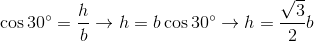Now that we have an expression for the height in terms of the base, we can plug this value into the equation for surface area and be left with only one unknown, the base that is equivalent to the edge length for which we want to solve: